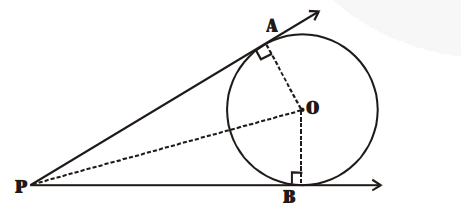# Prove that the angle between the two tangents drawn from an external point

Question:

Prove that the angle between the two tangents drawn from an external point to a circle is supplementary to the angle subtended by the line-segment joining the points of contact at the centre.

Solution:

Let PA and PB be two tangents drawn from an external point P to a circle with centre O.Now, in right $\Delta \mathrm{OAP}$ and right $\Delta \mathrm{OBP}$, we have

$\mathrm{PA}=\mathrm{PB}$  [Tangents to circle from an external point]

$\mathrm{OA}=\mathrm{OB}$ [Radii of the same circle]

OP = OP [Common]

$\Delta \mathrm{OAP} \cong \Delta \mathrm{OBP} \quad[$ By SSS congruency $]$

$\therefore \angle \mathrm{OPA}=\angle \mathrm{OPB} \quad[$ By C.P.C.T. $]$

and $\angle \mathrm{AOP}=\angle \mathrm{BOP}$

$\Rightarrow \angle \mathrm{APB}=2 \angle \mathrm{OPA}$ and $\angle \mathrm{AOB}=2 \angle \mathrm{AOP}$

But $\angle A O P=90^{\circ}-\angle O P A$

$\Rightarrow 2 \angle \mathrm{AOP}=180^{\circ}-2 \angle \mathrm{OPA}$

$\Rightarrow \angle A O B=180^{\circ}-\angle A P B$

$\Rightarrow \angle \mathrm{AOB}+\angle \mathrm{APB}=180^{\circ}($ Proved $)$